# An anti-aliasing filter for 24-bit ADCs

For many ADC applications, placing a simple RC filter at the buffer input provides adequate anti-aliasing filtering. For applications requiring higher order filters, active filters are often used. The active components in such a filter must have sufficient bandwidth, fast stability, low noise, and low offset to not corrupt the signal until it reaches the ADC.

The LTC6363 is a differential op amp optimized for driving low power SAR ADCs. The LTC6363 provides 500 MHz GBW, 780 ns stable to 4 ppm, and 2.9 nV/√Hz and 100μV maximum offset voltage.

Figure 1 shows a 30kHz third-order filter using the LTC6363, which is optimized for use with the 1.5Msps/2Msps low-power SARADC LTC2380-24 and has an integrated digital filter. The LTC2380-24 averages from 1 to 65536 conversions in real time, improving signal-to-noise ratio (SNR). Both inputs of this circuit can be driven differentially over a ±2.5 Vpp signal range, or one input can be grounded and the other input can be driven with signals up to ±5 Vpp.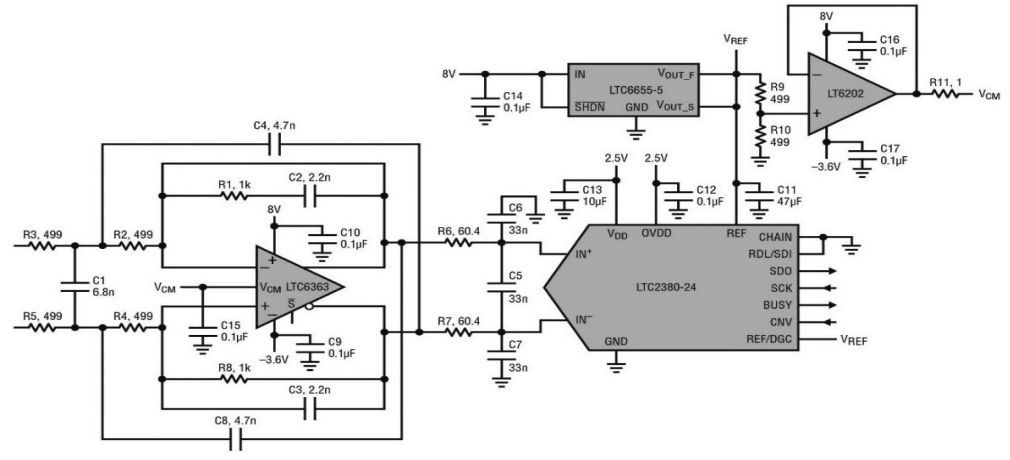Figure 1.30kHz third-order filter driver 24-bit ADC LTC2380-24

Figure 2 shows the frequency response of the filter and ADC together with a sampling rate of 1.5 Msps and the number of conversions (N) for the average is set to 1 and 8.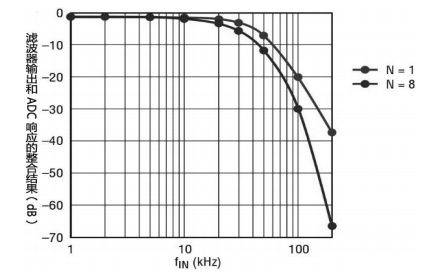Figure 2. Frequency response of the filter and ADC together

Figure 3. is a screenshot of PScope showing the FFT, SNR, and THD for the circuit shown in Figure 1 at N = 1.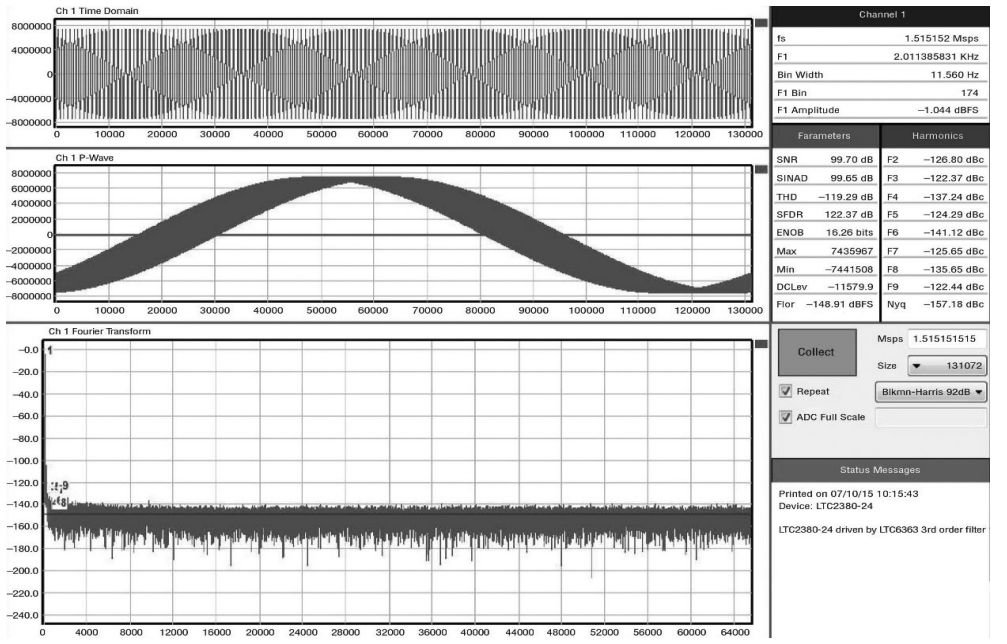Figure 3. The PScope screenshot shows the FFT, SNR, and THD for the circuit shown in Figure 1 at N=1.

Figures 4 and 5 show the THD and SNR as a function of input frequency for the circuit shown in Figure 1 at N = 1 and 8. When the input frequency is less than a few kHz, the performance of SNR and THD is close to the typical number in the datasheet. As the input frequency increases beyond a few kHz, the THD decreases smoothly.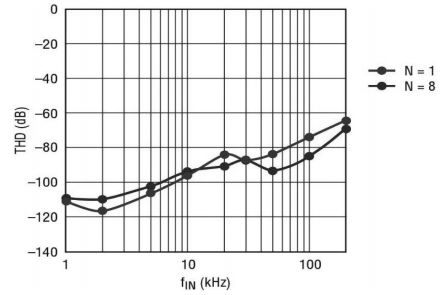Figure 4. Total harmonic distortion of the circuit shown in Figure 1 as a function of input frequency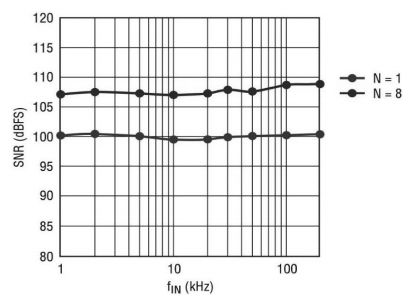Figure 5. Signal-to-noise ratio of the circuit shown in Figure 1 as a function of input frequency